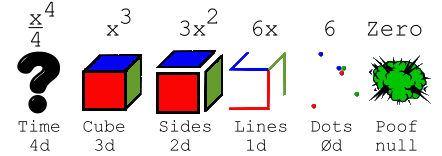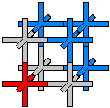Properties of Energy & Matter by Cranwell
 Nothing is Solid. Space is not Empty. Everything is Connected.

 Font Size A A A

 by James Clifford Cranwell 3/27/98 Part I ... Spatial DimensionalityContrary to popular belief... nothing is even remotely solid. At the sub atomic level it is well known the nucleus radius to electron orbital ratio is one hundred thousandth.
That makes the volumetric or spatial difference one quadrillionth = 10-15 = ( .000 000 000 000 001 ) . This ratio is approximately the same size as a spherical dot (tittle) above the  letter " i " (the proton) on the fifty - yard line in a football stadium (the orbital) everything else is empty space. That's 99.9999999999999 % empty space.
So, if we think of or visualize a huge sphere the size of a stadium (a small moon for instance), in reality the amount of actual continuous mass (just nuclei)
 (4/3)Pi 13 ---------------- (4/3)Pi 100,0003 = 1 --------------------- 1,000,000,000,000,000 = one  quadrillionth
is equivalent to a solid dot above the " i " made of only protons cut up into one quadrillion times 1,000 billion billion pieces and evenly dispersed. That's how many (one quadrillion) "i" dots would fill the moon sized sphere (ignoring sphere packing) times the number of atomic radii (1,000 billion billion) it would take to fill every "i" dot.
That's why neutrinos can zip right through anything completely unfettered and why the moon is only there when someone is looking at it... if we couldn't see vibrations of electron energy, the moon would be completely unnoticed.
It has the same amount of literally solid "stuff" as a spherical tittle.

Quantum Math

When we differentiate, we get the instantaneous change in whatever equation or shape we consider. It's easy to visualize because the starting equation or figure, for instance a 3-dimensional cube ( y = x3 ), gets lowered down a degree in power or dimension. i.e. ( y' = 3x2) this can be equated to three planar two dimensional sides of the cube or the instantaneous change needed to increase the length, width and height (volume) of the cube. So, this means to instantaneously increase volume, tack on area to half the outside surface. Taking this one step furtherwe arrive at ( y'' = 6x ). This is simply six one dimensional lines or the instantaneous change needed to increase the length and width (area) of the three planes. Every plane needs two lines (length and width) to increase its area. Three planes times two lines equals six lines total.
Now it gets even easier... six points ( y''' = 6 ) are the instantaneous change needed to increase six lines in length (notice the six points are zero-dimensional). Now we arrive at ( y (4 ) = 0 ) ...this is the instantaneous change needed to take the six points out of existence.
A big problem occurs when we try to integrate something cubic ( y = x3 ) into the fourth dimension, in this case ( integral y ). We have an exact mathematical representation of it ( x 4 /4 ) and if x = 1 we know this is equal to 1/4's worth of fourth dimensional volume (tesserarea?). But, what shape is it? Is it a snapshot in time? Is it an hypercube? Is it a mysterious visitor from the fourth dimension?

The Cube isn't Solid. Nothing is SolidRemember, nothing is even remotely solid. So you will rack your brain trying to visualize the integration of a solid or in this case an actual misconception.   The mysterious fourth dimensional shape is a tetrahedral axes shaped particle group of higher density.
Any one dimensional object is a line. Any two dimensional object is a plane. But that's a slice of a supposed cube, and can't be thought of as for instance a sheet of paper. Because, if we integrate enough of them into a stack, we have a solid cube of paper and by now we know... Nothing is Solid.
The cube can't be solid. So that form of thinkingis simply wrong (note: The one dimensional line would also have to be in segments, and although we can actually integrate lines into a plane ... the lines in this case are never arranged parallel so they won't form a continuous plane that could then be integrated into a stack)...

The cube isn't solid but since it is there, it must be made of something. If we call the basic unit of whatever the cube is comprised of a particle. The particle must be capable of conveying information, for instance electro - magnetic vibrations. And since there are different frequencies and / or strengths of vibrations with multiple simultaneous combinations, a zero-dimensional single point particle would be incapable of achieving this. It can spin or move or remain at rest but there is no chance of Simultaneity or vibrations. The next possible alternative is the one dimensional line or string (any intrinsic universal characteristic will always be the simplest and at the same time most efficient option).
The string seems to be the shape of choice in this case. On a musical instrument, a violin for instance, the string can convey a multitude of vibrations, tones and harmonics. This means there can be a lot of simultaneous information transmitted along a one dimensional string. There is no need to attempt theoretical construction of a particle made of two dimensional planes because... you don't keep looking for the answer after you have found it. And, if we integrate enough of the planes into a stack, we would have a solid. Nothing is Solid.

Particle Integration:

 x4/4 = 1/4 tessarea = 4 axis (? time)   x3 = 1 volume = 3 axis x 1  3x2 = 3 area   = 2 axis x 3   6x = 6 lines  = 1 line x 6
So, the basic building blocks of particle construction must be line segments or strings. It's their arrangement that makes all the difference. The basic unit of the x 3 variety is the X-Y-Z axes shaped particle. This is three strings joined at their centers. If in this example x = 1, then the three axis will each be 1 in length (X, Y and Z are 1 long) with a total length of Three with a cubic volume of 1. All widths are infinitesimal. When we differentiate x3 we get 3x2this is 3 plus signs or XY axes shaped particles that each have 2 axis with a total length of 6 and an area of 3, that makes 6 axis with 1 length each. This is also the correct amount to add one quadratic particle XY to every axis of the original differentiated particle giving the XYZ an instantaneous change.So, working this in reverse we see that as we integrate into a higher power it changes the shape by adding an axis and it shrinks in size (area, volume) giving it a greater density. So the fourth dimensional object is composed of tetrahedral axes shapes. In this example 4 axis with total length 4 and a fourth dimensional volume (tesserarea?) of one-fourth (1/4)
f (1) = x4 /4 = 1/4 ... that's 4 axis, each one 1 in length. More particles are needed to fill any volume because of the tighter pack, ergo higher density. Now it is easy to see... in a field of 2-D ( XY ) particles we can only traverse horizontally and vertically. When we bump this up one dimension into 3-D we also have the toward and away axis. Alas, we still can't move on a diagonal, for that is reserved for the higher dimensions.
Now it's a piece of cake to see how any length, for instance 1/(10 * √26 - 1)c, divided up correctly can be a direct representation of a particle(s).

Spatial dimension is directions.

An Abbott Flatlander from Flatland living on a two dimensional plane would actually be living on an infinity of dimensionsif he can turn or move through every angle or vector direction on the supposed plane. The way this actually works is by using axial directions as dimension.
An actual working two dimensional model of space would be an infinite array of 2-D axis shaped particles arranged in a plane with the negative or expansive force vibrating through their continuum (matrix). In this 2-D model light is coerced into traveling in straight lines in only two directions (This model can also warp or flex, forcing the curvature of light). If you took enough 2-D particles and curved and connected them into a spherical surface shape, it would be misinterpreted as 3-D.
The actual 2-D electron is pulling this same prank by orbiting spherically and mimicking a 3-D solid. Nothing is actually 3-D and / or solid. Now that we know the basic workings of particles at the quantum level and we know vibrations occur in every possible direction, a 3-dimensional particle will not sufficiently transmit vibrations along a diagonal.
So, 3-D doesn't work. The particle capable of angular conveyance must be of higher dimension and have the most efficient shape to pack space. It turns out to be a particle with 10-dimensions or ten axes. In the ten dimensional dodecahedral matrix light is actually forced into zigzags.
This axial concept allows for an actual visual of higher dimensions. If we integrate a supposed three-dimensional cube into the fourth dimension the result isn't a snapshot of the cube in the fourth dimension, it is a tetrahedron with an increased density field matrix (tetrahedral).
You have to remember... nothing is 3-D and / or solid.
A proton is 0-D (zero-D), the electrons orbiting it are 2-D, we're seeing this whole configuration through a 10-D field.
So if you want to bump up one dimension higher than the dimension you actually see things in, you're going to have to contemplate a mysterious visitor from the eleventh dimension (not the fourth).
If you want to bump up something in dimension until it is actually solid... you're on your way to creating your very own neutron star with a contiguous proton/neutron pack, We're all in trouble if we get a visit from one of them.

Quantum Weirdness:

Everything might be actually happening very, very slowly. Since everything involved in the continuum structure is completely controlled or regulated at the speed of light (including Stars, Planets, any type of measuring device, Plants, Animals, Humans and everything else, excluding for instance neutrinos), we have no way of knowing what speed things are really happening.
It's like being a character in a movie, you are just film and you are trying to find out what speed the projector is running.
If you could take a step out and away, literally remove yourself from everything and look back at what's happening, The movie might run fast, slow, even stop and start and no one would be the wiser. There would be no way for the people still in the movie to discern this. The movie would be their whole universe, their very existence. The speed the projector is running is the speed their lives are happening.
The Speed of light and all particle interactions might be traveling or happening at the pace of an Escargot (snail) but our brains are using the same speed vibration set by this cosmic speedometer so we'll never know.

 Properties of Energy & Matter * part II *

Goddess401 Home Page    admin@gootar.com     https://xulfrepus.neocities.org/